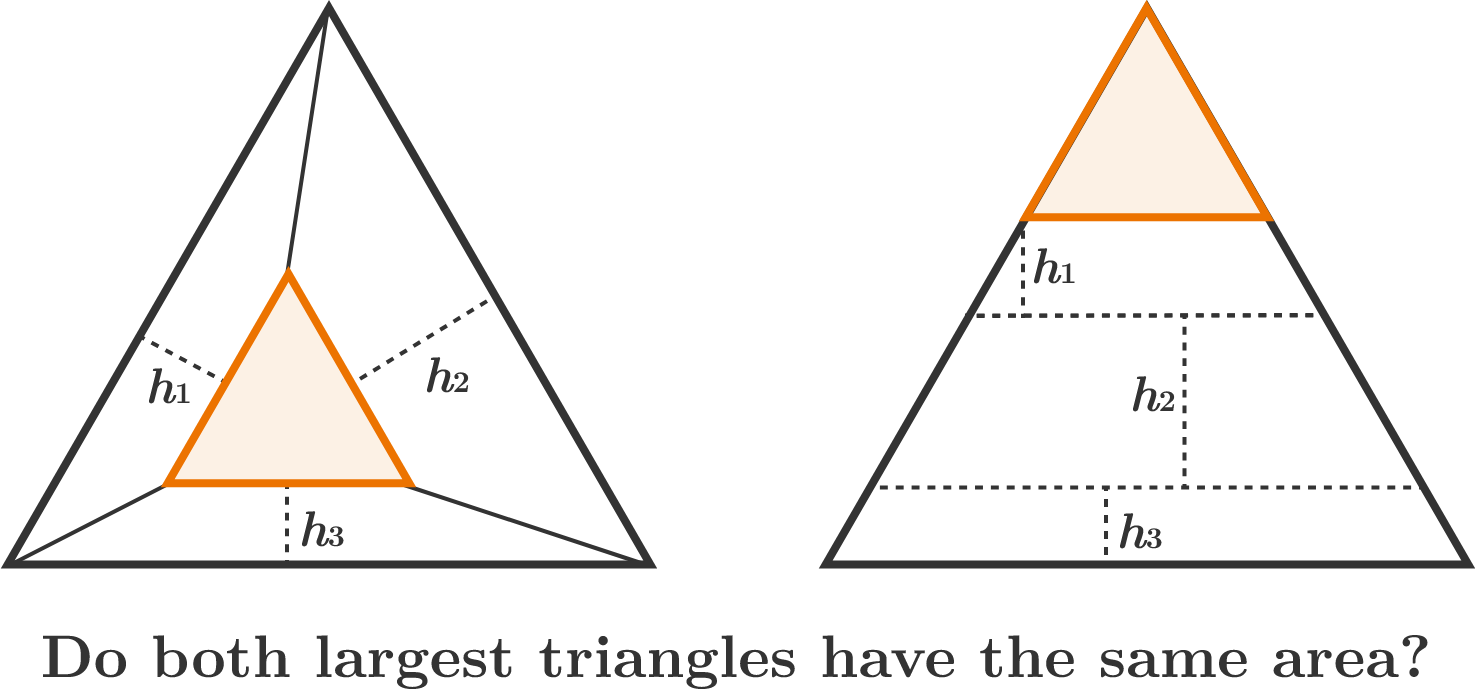# One Height Over Another

Geometry Level 3Two identical, orange equilateral triangles are each positioned in a large, black triangle such that

• in the left diagram, the corresponding sides of the two triangles are parallel to each other;
• in the right diagram, the orange triangle fits in the top corner of the black triangle.

Then, we find that three trapezoids with respective heights $h_1, h_2, h_3$ can be formed in each black triangle, as shown.


True or False?

The two black triangles have equal areas.

×